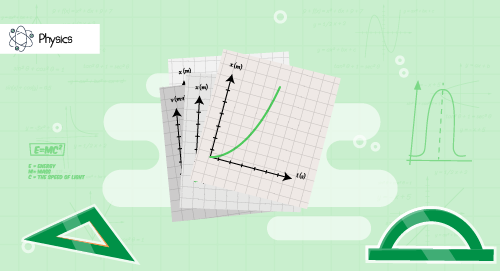#### Need Help?

Get in touch with usSAT/ACT

# Graphs of motion

Physics

This module covers the basic terms associated with the motion of an object such as displacement, distance, speed, velocity, acceleration and time with their mathematical representations, plotting the velocity-time graphs and position-time graph, calculating velocity from the position-time graphs and acceleration and displacement from the velocity-time graphs. It also explains the 3 equations of motion which connect all the attributes of moiton of a body. These equations of motion can be used to solve numerical problems and derive conclusions.

##### Course Duration
•3 Weeks5 Sessions
•50 mins per session
##### Tutor## What you'll learn

• 1

To define various terms associated with the motion of an object such as the velocity, speed, distance, displacement, acceleration and time.

• 2

To plot position-time graphs and velocity-time graphs of the motion of a body.

• 3

To identify patterns of the v-t and s-t graphs so as to derive conclusions about the type of motion or the pattern of variation of speed, velocity, acceleration, displacement and distance w.r.to time.

• 4

To calculate velocity from the s-t graphs and acceleration and displacement from the v-t graphs.

• 5

To understand the 3 equations of motion.

• 6

To use the equations of motion to solve mathematical problems and derive conclusions from the result.

## Skills you'll learnPlotting graphsInfering graphsStatistical skillsMathematical skillsProblem solvingAnalytical skills